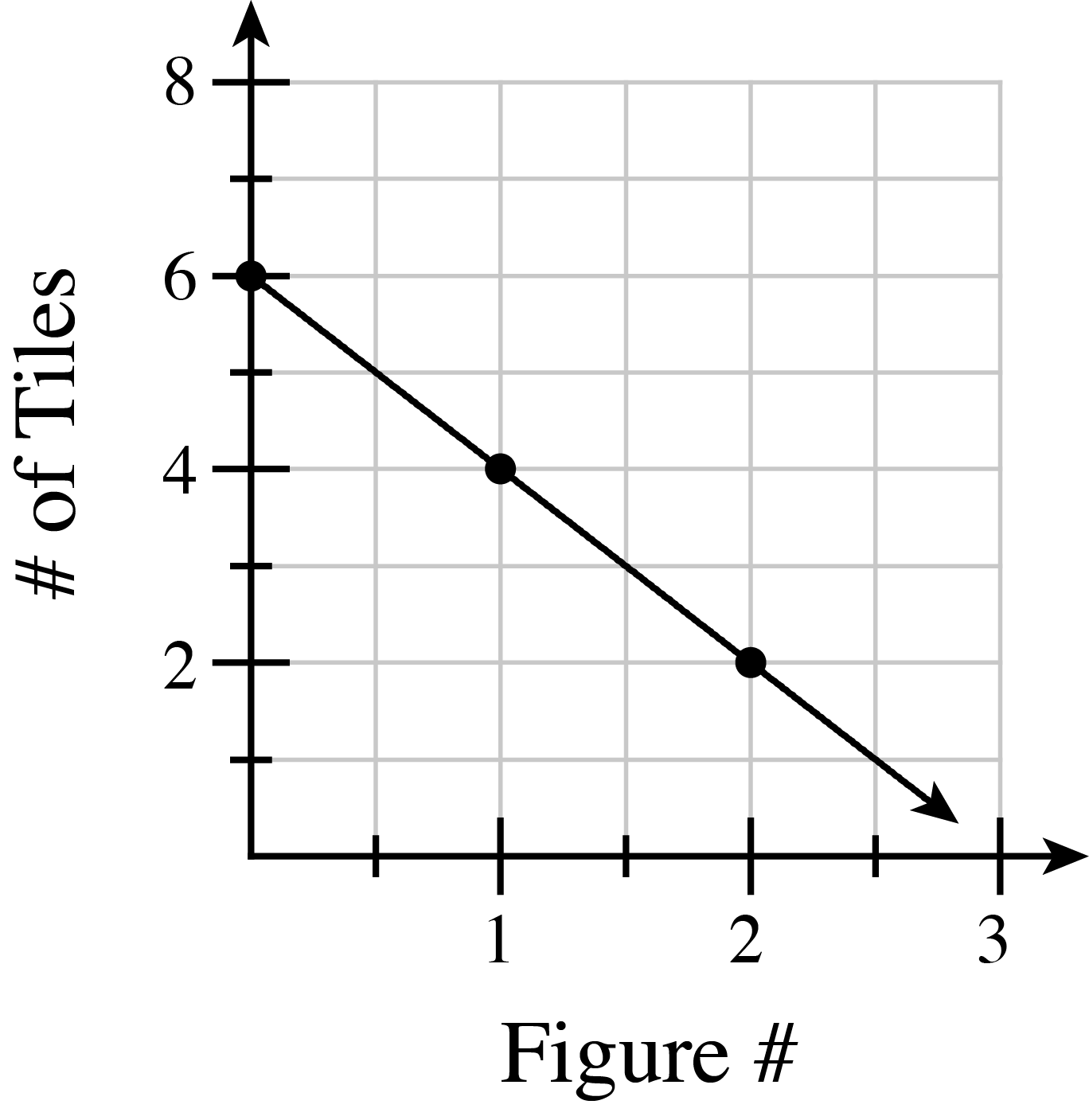### Home > CC3MN > Chapter 9 > Lesson 9.2.2 > Problem9-116

9-116.This problem is a checkpoint for multiple representations of linear equations. It will be referred to as Checkpoint 9B.

For each situation given below, complete the Representations of Patterns Web by finding the missing $x→y$ table, graph, and/or rule. Since there are many possible patterns, it is not necessary to create one.

1.  tile $\space$ tile $\space$ tile $\space$ tile $\space$

Figure $1$

 tile $\space$ tile $\space$ tile $\space$ tile $\space$ tile $\space$ tile $\space$

Figure $2$

 tile $\space$ tile $\space$ tile $\space$ tile $\space$ tile $\space$ tile $\space$ tile $\space$ tile $\space$

Figure $3$

1. $y=−3x+7$

1.  $x$ x column 1 $0$ x column 2 is blank. x column 3 is blank. x column  4 $3$ x column 5 is blank.$\$ $y$ y column 1 $1$ y column 2 is blank. y column 3 is blank. y column 4 $10$ y column 5 is blank.
1.Check your answers by referring to the Checkpoint 9B materials located at the back of you book.

Ideally, at this point you are comfortable working with these types of problems and can solve them correctly. If you feel that you need more confidence when solving these types of problems, then review the Checkpoint 9B materials and try the practice problems provided. From this point on, you will be expected to do problems like these correctly and with confidence.

Answers and extra practice for the Checkpoint problems are located in the back of your printed textbook or in the Reference Tab of your eBook. If you have an eBook for Core Connections, Course 3, login and then click the following link:  Checkpoint 9B: Multiple Representations of Linear Equations